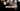# Leetcode | Solution of Ransom Note in JavaScript

May 15th, 2020
|

In this post, we will solve Ransom Note from leetcode and compute it's time and space complexities. Let's begin.

# Problem Statement

The question can be found at leetcode Ransom Note problem.

The problem states that we are given two strings, a magazine, and a ransom note. We need to find if the string ransom note can be created from the magazine.

# Constraints and challenges

• Both the strings only contain lower case characters
• Typing those big variable names can be a pain# Solution

The approach is very simple. We declare a map and save all the characters from the magazine with its count in the map.

Next, we loop over the ransom note and see if the map contains enough characters to create out ransom note string.

It's a very simple question with a fancy name, thats all.

We have discussed the approach, I urge you to go ahead on leetcode and give it another try.If you are here, it means something went wrong in implementation or you are just too lazy. In any case, let's see a simple implementation of the above logic.
``````var canConstruct = function (ransomNote, magazine) {
const map = new Map();

for (let i = 0; i < magazine.length; i++) {
if (map.has(magazine[i])) {
map.set(magazine[i], map.get(magazine[i]) + 1)
} else {
map.set(magazine[i], 1)
}
}

for (let i = 0; i < ransomNote.length; i++) {
if (!map.has(ransomNote[i]) || map.get(ransomNote[i]) === 0) {
return false;
}
map.set(ransomNote[i], map.get(ransomNote[i]) - 1)
}

return true;
};``````

Nothing fancy in the code, just simple logic, and big variable names.

Here are the stats on submission

``````Status: Accepted
Runtime: 172ms
Memory: 37.2MB``````

## Time and space complexity

### Time complexity

We are looping over both the strings once, so time complexity would be O(n).

### Space complexity

We are using extra space for the map. So space complexity is linear, O(n).

# Summary

So, we solved the Ransom Note problem by using two pointer method and calculated the time and space complexities.

I hope you enjoyed solving this question. This is it for this one, complete source code for this post can be found on my Github Repo. Will see you in the next one.